# RD Sharma Class 8 Solutions Chapter 9 Linear Equations in One Variable Ex 9.2

In this chapter, we provide RD Sharma Class 8 Solutions Chapter 9 Linear Equations in One Variable Ex 9.2 for English medium students, Which will very helpful for every student in their exams. Students can download the latest RD Sharma Class 8 Solutions Chapter 9 Linear Equations in One Variable Ex 9.2 Maths pdf, free RD Sharma Class 8 Solutions Chapter 9 Linear Equations in One Variable Ex 9.2 book pdf download. Now you will get step by step solution to each question.

### RD Sharma Solutions for Class 8 Chapter 9 Linear Equations in One Variable Ex 9.2Download PDF

Solve each of the following equations and also check your result in each case :
Question 1.
2x+53 = 3x – 10
Solution:
2x+53 = 3x–101
By cross multiplication
⇒ 2x + 5 = 3 (3x – 10)
⇒ 2x + 5 = 9x – 30
⇒ 5 + 30 = 9x – 2x (By transposition)
⇒ 35 = 7x
⇒ x = 5Question 2.
a–83 = a–32
Solution:Question 3.
7y+25 = 6y–511
Solution: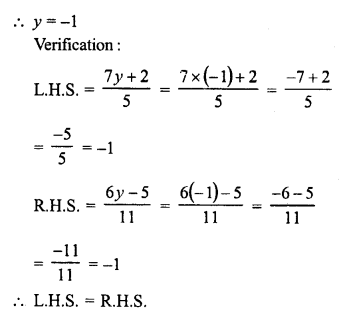Question 4.
x – 2x + 2 – 163 x + 5 = 3 – 72 x.
Solution:Question 5.
12 x + 7x – 6 = 7x + 14
Solution: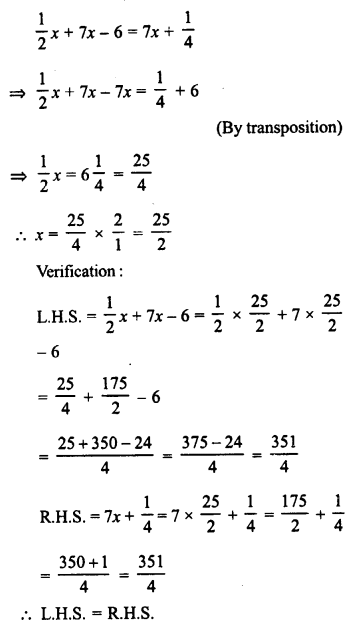Question 6.
34 x + 4x = 78 + 6x – 6
Solution: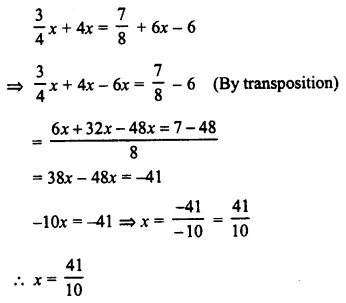Question 7.
72 x – 52 x = 203 x + 10
Solution:
72 x – 52 x = 203 x + 10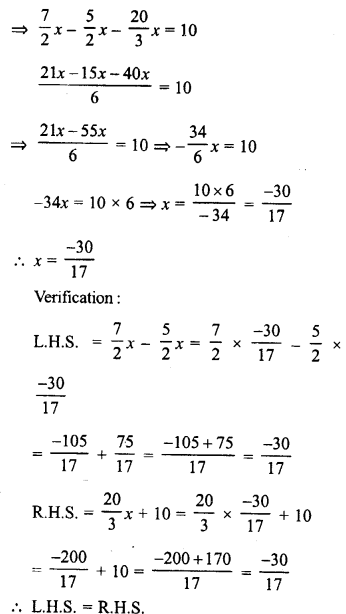Question 8.
6x+12 + 1 = 7x–33
Solution: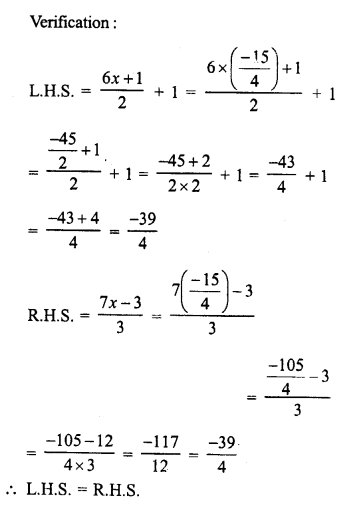Question 9.
3a–23 + 2a+32 = a + 76
Solution:Question 10.
x – x–12 = 1 – x–23
Solution: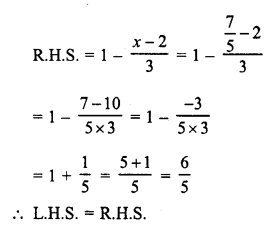Question 11.
3×4 – x–12 = x–23
Solution: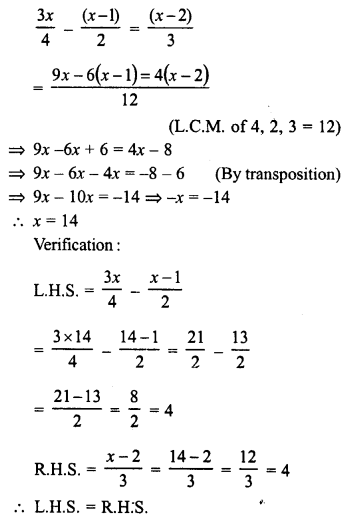Question 12.
5×3 – x–14 = x–35
Solution:
5×3 – x–14 = x–35Question 13.Solution:Question 14.Solution:Question 15.Solution:Question 16.
0.18 (5x – 4) = 0.5x + 0.8
Solution:Question 17.Solution: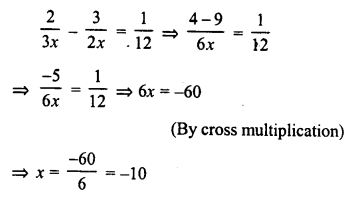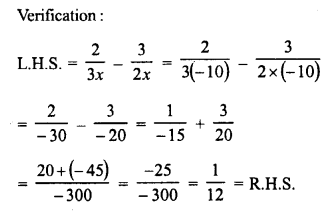Question 18.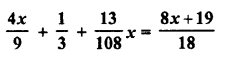Solution:Question 19.Solution:Question 20.Solution: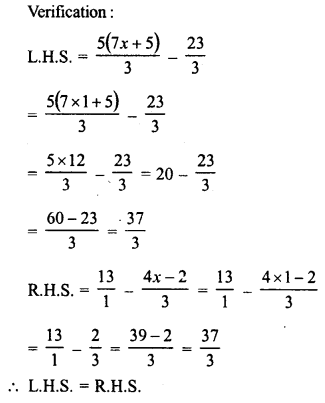Question 21.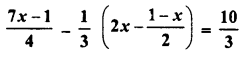Solution: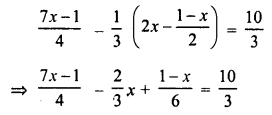Question 22.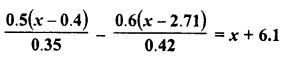Solution: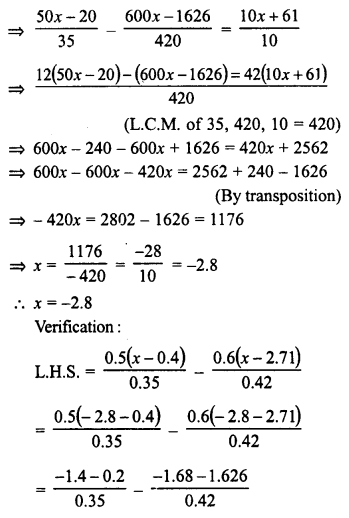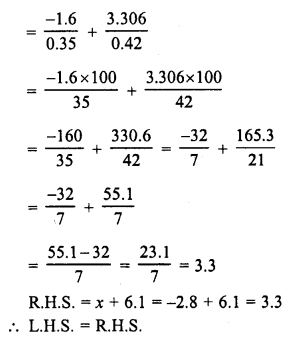Question 23.Solution:Question 24.
(3x – 8) (3x + 2) – (4x – 11) (2x + 1) = (x – 3) (x + 7)
Solution:
(3x – 8) (3x + 2) – (4x – 11) (2x + 1) = (x – 3) (x + 7)
⇒ (9x² + 6x – 24x – 16) – (8x² + 4x – 22x – 11) = x² + 7x – 3x – 21
⇒ 9x² + 6x – 24x – 16 – 8x² – 4x + 22x + 11 = x² + 4x – 21
⇒ 9x² – 8x² – x² + 6x – 24x + 22x – 4x – 4x = -21 + 16 – 11
⇒ 28x – 32x = -32 + 16
⇒ -4x = -16
⇒ x = 4
Verification:
L.H.S. = (3x – 8) (3x + 2) – (4x – 11) (2x + 1)
= (3 x 4 – 8) (3 x 4 + 2) – (4 x 4 – 11) (2 x 4 + 1)
= (12 – 8) (12 + 2) – (16 – 11) (8 + 1)
= 4 x 14 – 5 x 9 = 56 – 45 = 11
R.H.S. = (x – 3) (x + 7) = (4 – 3) (4 + 7) = 1 x 11 = 11
L.H.S. = R.H.S.

Question 25.
[(2x + 3) + (x + 5)]² + [(2x + 3) – (x + 5)]² = 10x² + 92
Solution:
[(2x + 3) + (x + 5)]² + [(2x + 3) – (x + 5)]² = 10x² + 92
⇒ (2x + 3 + x + 5)² + (2x + 3 – x – 5)² = 10x² + 92
⇒ (3x + 8)² + (x – 2)² = 10x² + 92
⇒ 9x² + 2 x 3x x 8 + 64 + x² – 2 x x x 2 + 4 = 10x² + 92
⇒ 9x² + 48x + 64 + x² – 4x + 4 = 10x² + 92
⇒ 9x² + x² – 10x² + 48x – 4x = 92 – 64 – 4
⇒ 44x = 24All Chapter RD Sharma Solutions For Class 8 Maths

—————————————————————————–

All Subject NCERT Exemplar Problems Solutions For Class 8

All Subject NCERT Solutions For Class 8

*************************************************

I think you got complete solutions for this chapter. If You have any queries regarding this chapter, please comment on the below section our subject teacher will answer you. We tried our best to give complete solutions so you got good marks in your exam.

If these solutions have helped you, you can also share rdsharmasolutions.in to your friends.